# Statistical modelling Introduction to probabilistic and statistical modelling of risk

## Overview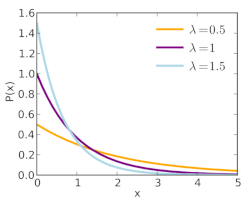Risk analysis is sometimes based on the analysis of data concerning a hazardous event, such as the occurrence of an earthquake, or the exceedance of a threshold. This analysis is based on statistical modelling, most often with computer tools. When the risk analyst puts her data scientist hat on, she collects data (measurements, observations) from various sources and inputs them into the computer, obtains a general overview of the data and its distribution, and builds a statistical model which attempts to reproduce properties of the underlying phenomena. After checking that the statistical model is a good fit for the observations, she can generate various risk metrics and quantify the level of uncertainty in the predictions.

Statistical modelling (or “data science” or “machine learning”, to use related and more trendy terms) is an important part of risk analysis and safety in various engineering areas (mechanical engineering, nuclear engineering), in the management of natural hazards, in quality control, and in finance.

This submodule is a part of the risk analysis module.

## Learning objectives

Upon completion of this submodule, you should be able to:

• Analyze data using descriptive statistics and graphical tools

• Fit a probability distribution to data (estimate distribution parameters)

• Express various risk measures as statistical tests

• Determine quantile measures of various risk metrics

• Build flexible models to allow estimation of quantities of interest and associated uncertainty measures

• Select appropriate distributions of random variables/vectors for random phenomena

## Course material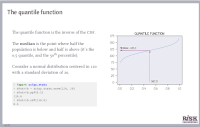Statistics and risk modelling with PythonLecture slides (PDF)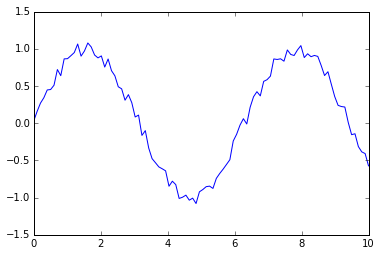Python notebook on basic statisticsDownload Python notebookRun notebook in MyBinderRun notebook in Google ColabPython notebook on coins and diceDownload Python notebookRun notebook in MyBinderRun notebook in Google Colab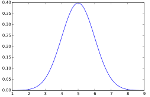Python notebook on probability distributionsDownload Python notebookRun notebook in MyBinderRun notebook in Google Colab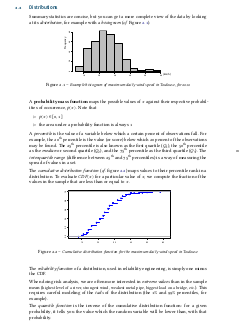Brief reminder on statisticsHandout (PDF)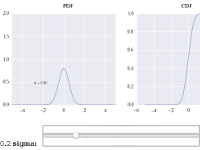Interactive examples of probability distributions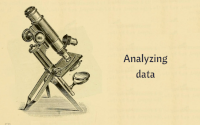Analyzing data with PythonLecture slides (PDF)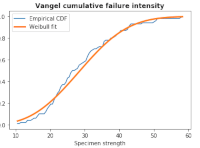Exercise (Python notebook) on descriptive statisticsDownload Python notebookRun notebook in MyBinderRun notebook in Google ColabPython notebook on simple descriptive statisticsDownload Python notebookRun notebook in MyBinderRun notebook in Google Colab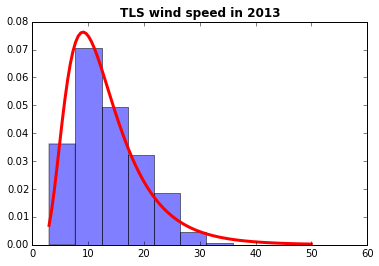Python notebook on analysis and curve fitting for weather dataDownload Python notebookRun notebook in MyBinderRun notebook in Google Colab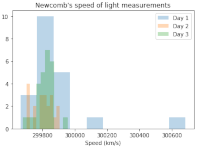Python notebook on analysis of speed of light measurementsDownload Python notebookRun notebook in MyBinderRun notebook in Google Colab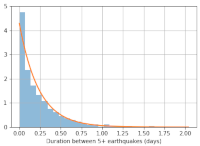Python notebook on analysis of earthquake dataDownload Python notebookRun notebook in MyBinderRun notebook in Google Colab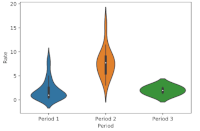Python notebook on Semmelweis’s work on risk reduction in hospitalsDownload Python notebookRun notebook in MyBinderRun notebook in Google Colab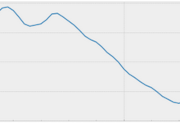Python notebook on intervention analysis using a hierarchical modelDownload Python notebookRun notebook in MyBinderRun notebook in Google ColabIn these course materials, applications are presented using the NumPy, SciPy and statsmodels libraries for the Python programming language. We have some material on getting started with Python that explains how to install Python on your computer or try out our computational notebooks using free online services.

## Other resources

We recommend the following sources of further information on this topic: